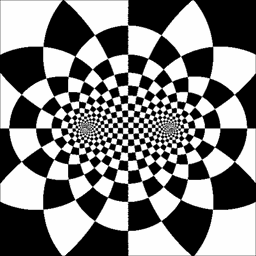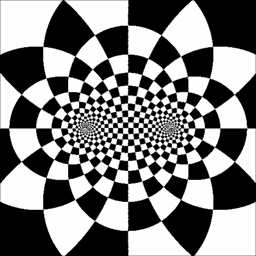## Wednesday, August 31, 2011

### Moebius transformation animated GIFs

Here are some animated GIFs that I created a few years ago with MATLAB.  These characterize the action of the four classes of Moebius transformations, mapping the complex plane to itself.  They were inspired by the illustrations and analysis in a section of Tristan Needham's excellent book Visual Complex Analysis:elliptichyperbolicloxodromicparabolic

So what's it all about?  A Moebius transformation is a mapping on the complex numbers of the form $M(z) = \frac{az + b}{cz + d},$ where $$a, b, c, d$$ are complex constants.  Moebius tranformations have many nice features: they are one-to-one and onto on the complex plane (extended with the addition of the "point at $$\infty$$"), they form a group under composition, they are conformal (angle preserving).  Interestingly, though conformality only implies that they map infinitesimal circles to other infinitesimal circles, Moebius transformations actually map circles of any size in the complex plane to other circles in the plane.  For a really nice exposition and proofs of these qualities, see Needham.

Fixed points on the plane under a Moebius transform will simply be solutions of $z = \frac{az+b}{cz+d},$ which is just quadratic in $$z$$.  So a (non-identity) Moebius transform will have at most two fixed points, or one when there is a repeated root.  Following Needham, for the remainder of this discussion we'll refer to the fixed points of a given Moebius transformation as $$\xi_+$$, $$\xi_-$$ in the two root case, or just $$\xi$$ in the repeated root case.

Most of the qualities above are readily observable in the animations: one or two fixed points, circles mapping to circles, one-to-one, easy to imagine extension to the whole plane.  Conformality is a little harder to see, but if you look closely you can see that the angles at each of the corners in these crazed checkerboards always remain 90°.

The GIFs were generated using a transform method.  Given a Moebius transformation with two fixed points (we will revisit the single fixed point case shortly), consider the additional Moebius transformation defined by $F(z) = \frac{z - \xi_+}{z - \xi_-}.$This will send $$\xi_+$$ to $$0$$, and $$\xi_-$$ to $$\infty$$.  We can now construct $\widetilde{M} = F \circ M \circ F^{-1},$ which is itself a Moebius transformation (since the Moebius transformations are a group under composition) and which will have fixed points at $$0$$ and $$\infty$$.  We can consider $$M$$ and $$\widetilde{M}$$ to be a transform pair; for any $$M$$ there is a corresponding $$\widetilde{M}$$ with fixed points at $$0$$ and $$\infty$$.

Now it turns out that Moebius transformations with fixed points at both $$0$$ and $$\infty$$ reduce to a particularly simple form — that of a single complex multiplication, i.e. just a dilation and/or rotation about the origin of the complex plane.  See Needham for exposition of this.  The elliptic, hyperbolic, and loxodromic classes of Moebius transformations turn out to be those whose corresponding $$\widetilde{M}$$ is a pure rotation, pure dilation, or general combination of the two, respectively.

To generate these GIFs, we decorate the complex plane on the $$\widetilde{M}$$ side like a circular checkerboard or dart board, and represent the action of a given class of $$\widetilde{M}$$ transformations by rotating it, dilating it, or a combination of both, e.g.:elliptic $$\widetilde{M}$$hyperbolic $$\widetilde{M}$$

On the $$M$$ side, we can pick fixed points $$\xi_+$$, $$\xi_-$$ wherever we like, and then derive the corresponding $$F$$ as above.  For each frame, we take each point on the $$M$$ side, map it through $$F$$ to find the corresponding point on the $$\widetilde{M}$$ side, and check that against a dynamic checkerboard model to see if the point should be black or white in this frame.

Returning to the repeated root, single fixed point case: we treat this similarly, but set $F = \frac{1}{z - \xi},$ which sends $$\xi$$ to $$\infty$$.  As before, it turns out that Moebius transformations of this form (repeated fixed point at $$\infty$$) reduce to a very simple form: this time, a pure translation.  The parabolic class of Moebius transformations are those whose corresponding $$\widetilde{M}$$ transformation is a pure translation.  To illustrate these, we use a translating dynamic checkerboard on the $$\widetilde{M}$$ side that looks like this:parabolic $$\widetilde{M}$$

All of this can be done quite succinctly in MATLAB, once it is understood what needs to be done.  Below is the MATLAB snippet which was used to generate these GIFs.  Commenting and uncommenting various lines chooses different $$F$$ and $$\widetilde{M}$$ side checkerboard models, resulting in the different outputs.

The first few lines create a 512x512 matrix of complex numbers, ranging from -3 to 3 on both the real and imaginary axes, to represent a portion of the complex plane.  This is then pre-mapped through an appropriate $$F$$.  The for loop iterates on each frame.  The dynamic checkerboard result is calculated as the xor of various versions of functions g1 and g2 operating over the premapped points.  Each frame is downsampled and written to disk as a separate file, then at the end all the frames are stitched into a movie.  I must then have used some non-matlab utility to convert the movies to animated GIFs, but I'm not sure what that was...

Z = -3:6/512:3-(6/512); Z = Z(ones(1,length(Z)),:); Z = complex(Z,Z'); %Z = 1 ./ Z; % one finite fixed point Z = (Z+1)./(Z-1); % two finite fixed points im = []; g1 = []; g2 = []; for frame = 1:30 g1 = mod(log(abs(Z))*4,2)<1; % radial, static % g1 = mod(log(abs(Z))*4+(frame-1)/15,2)<1; % radial, dynamic % g1 = mod(real(Z)+(frame-1)*.8/30,.8)<.4; % vertical, dynamic % g2 = mod(angle(Z), pi/6)<(pi/12); % circumferential, static g2 = mod(angle(Z)+(frame-1)*pi/180, pi/6)<(pi/12); % circumferential, dynamic % g2 = mod(imag(Z),.8)<.4; % horizontal, static im(:,:,1,frame) = imresize(xor(g1,g2), .25, 'bilinear'); imwrite(im(:,:,1,frame), sprintf('frame%.2d.bmp', frame)); end mov = immovie(255*im,gray(255)); movie(mov,50);

#### 1 comment:

1.